Electron. J. Diff. Equ., Vol. 2013 (2013), No. 70, pp. 1-8.

### Point rupture solutions of a singular elliptic equation Huiqiang Jiang, Attou Miloua

Abstract:
We consider the elliptic equation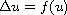in a region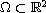, where f is a positive continuous function satisfying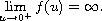Motivated by the thin film equations, a solutionis said to be a point rupture solution if for some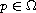,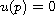and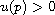in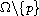. Our main result is a sufficient condition on f for the existence of radial point rupture solutions.

Submitted May 2, 2012. Published March 13, 2013.
Math Subject Classifications: 49Q20, 35J60, 35Q35.
Key Words: Thin film; point rupture; radial solution; singular equation.

Show me the PDF file (206 KB), TEX file, and other files for this article.Huiqiang Jiang Department of Mathematics, University of Pittsburgh Pittsburgh, PA 15260, USA email: hqjiang@pitt.edu Attou Miloua Department of Mathematics, University of Pittsburgh Pittsburgh, PA 15260, USA email: atm33@pitt.edu